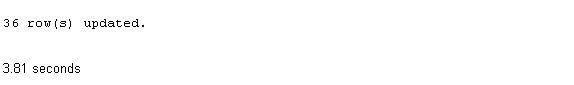# SQL update columns with arithmetical expression

In this page, we are going to discuss how to change the data of the columns with the SQL UPDATE statement using an arithmetical expression.

Example:

Sample table: neworder

To change the value of 'advance_amount' column with a new value as specified -

1. 'ord_amount'*10,

the following SQL statement can be used:

SQL Code:

``````UPDATE neworder

Output:## SQL update columns with arithmetical expression and where

In the following, we are going to discuss how to change the data of the columns with the SQL UPDATE statement using arithmetical expression and SQL WHERE clause.

Example:

Sample table: neworder

To update the value of 'advance_amount' with following conditions -

1. new value for 'advance_amount is 'ord_amount'*10,

2. 'ord_date' must be greater than '01-Aug-08',

the following SQL statement can be used:

SQL Code:

``````UPDATE neworder
WHERE ord_date>'01-Aug-08';``````

## SQL update columns with arithmetical expression and boolean 'AND'

In the following, we are going to discuss how to change the data of the columns with the SQL UPDATE statement using arithmetical expression and SQL WHERE clause and boolean operator AND.

Example:

Sample table: customer1

To change the value of 'outstanding_amt' of 'customer1' table with following conditions -

1. modified value for 'outstanding_amt' is 'outstanding_amt'-('outstanding_amt'*.10),

2. 'cust_country' must be 'India',

3. and 'grade' must be 1,

the following SQL statement can be used :

SQL Code:

``````UPDATE customer1
SET outstanding_amt=outstanding_amt-(outstanding_amt*.10)
``````

## SQL update columns with arithmetical expression and comparison operator

In the following, we are discussing, how to change the data of the columns with the SQL UPDATE statement using arithmetical expression and COMPARISON operator.

Example:

Sample table: neworder

To change the value of 'advance_amount' of 'neworder' table with the following condition -

1. modified value for 'advance_amount' is 'ord_amount'*.10,

2. 'ord_date' must be greater than '01-Aug-08',

3. and 'ord_date' must be less than '01-Dec-08',

the following SQL statement can be used:

SQL Code:

``````UPDATE neworder
WHERE ord_date>'01-Aug-08' AND ord_date<'01-Dec-08';
``````

See our Model Database

Here is a new document which is a collection of questions with short and simple answers, useful for learning SQL as well as for interviews.

Practice SQL Exercises

﻿

## SQL: Tips of the Day

SQL MAX of multiple columns?

```SELECT
CASE
WHEN Date1 >= Date2 AND Date1 >= Date3 THEN Date1
WHEN Date2 >= Date1 AND Date2 >= Date3 THEN Date2
WHEN Date3 >= Date1 AND Date3 >= Date2 THEN Date3
ELSE                                        Date1
END AS MostRecentDate
```

Ref: https://bit.ly/3N5pffU